• 本片博客主要介绍计算机网络的主要分类方法以及计算机网络拓扑结构常用术语，计算机网络常用拓扑分类，无线局域网拓扑分类。计算机网络主要分类方法：计算机网络拓扑常用术语、拓扑分类以及无线局域网拓扑分类： ...
本片博客主要介绍计算机网络的主要分类方法以及计算机网络拓扑结构常用术语，计算机网络常用拓扑分类，无线局域网拓扑分类。

计算机网络主要分类方法：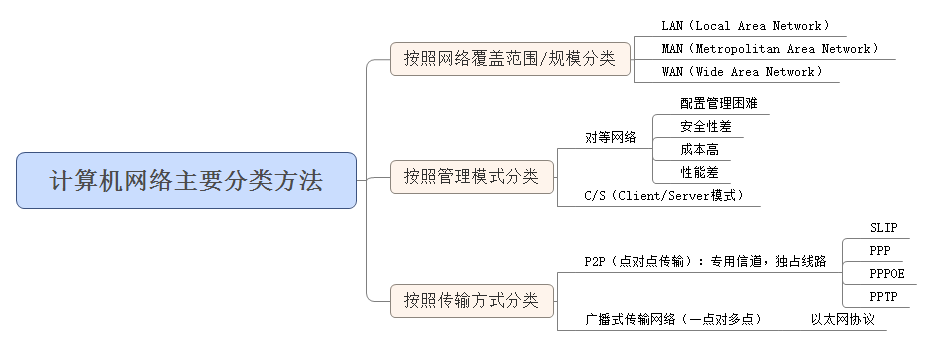计算机网络拓扑常用术语、拓扑分类以及无线局域网拓扑分类： 其中对于无线局域网拓扑分类主要是就管理模式而言。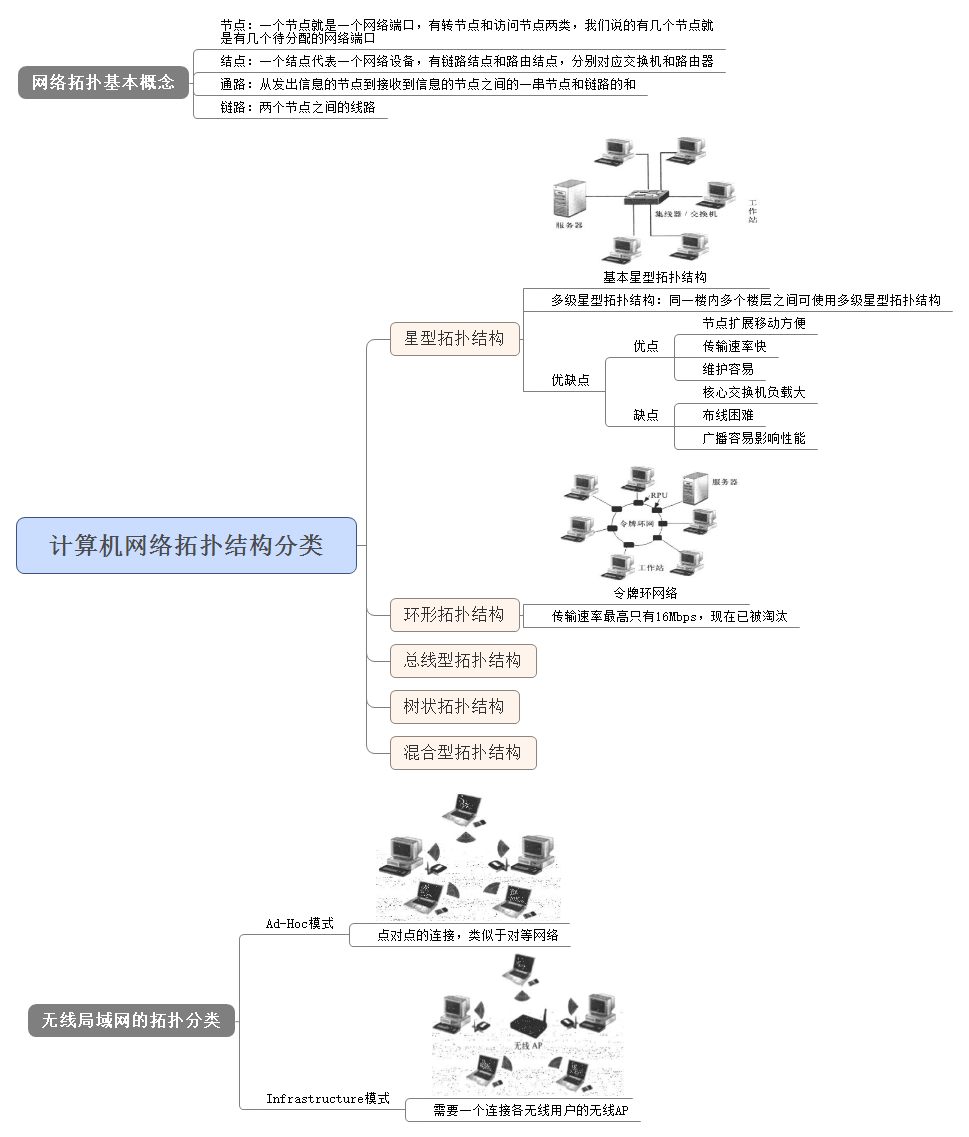图片以及Xmind文件下载地址：点我
展开全文计算机网络 无线局域网拓扑结构
• 模式识别是指：对于输入的模式x∈R，将其分类到它属于的类别y∈[1,...,c]的方法。c表示类别的数目。 2.最小二乘分类 首先考虑2类别分类问题y∈{+1，-1}.这种情况下，分类器的学习问题可以近似地定义为取值为+1...
1.前言

接下来主要介绍有关模式识别的算法。不可否认的是，在全民AI时代，PR的重要性越来越凸显了。

模式识别是指：对于输入的模式x∈R，将其分类到它属于的类别y∈[1,...,c]的方法。c表示类别的数目。

2.最小二乘分类

首先考虑2类别分类问题y∈{+1，-1}.这种情况下，分类器的学习问题可以近似地定义为取值为+1、-1的二值函数问题，如下图所示：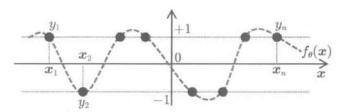函数近似分类的问题
像这样的二值函数，可以使用最小二乘法进行与回归算法相同的学习。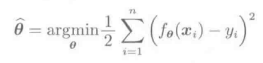测试模式x所对应的类别y的预测值y'是由学习后的输出结果的符号决定的：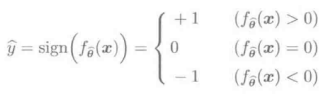注意：f=0是指实际上不怎么会发生的事件，也就是小概率事件。
像这样，把分类问题看成函数的近似问题，通过在分类器得构造中采用最小二乘法，就可以对前面我们学习到的最小二乘学习法进行拓展并灵活应用了。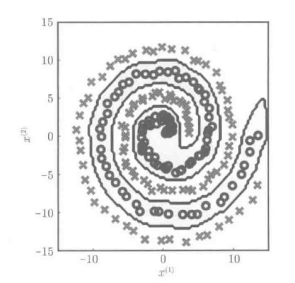上图展示的是使用L2约束的最小二乘学习进行模式识别的例子。虽然很复杂，但是也完成了分类。
还是先复习一下高斯核模型：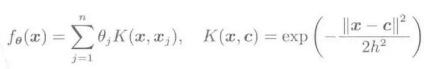注意：线性判别分析中，当正负两样本的模式都服从协方差矩阵相同的高斯分布时，可以获得最佳的泛化能力。

3.0/1损失和间隔

如下式所示：分类问题中使用函数的正负符号来进行模式判断，函数值本身的大小并不是那么的重要。因此，分类问题中如果应用如下式所示的0/1损失的话，应该会比L2损失得到更好的结果：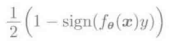上式0/1损失与下式是等价的：当分类错误的时候，函数结果为1；当分类正确的时候，函数结果为0.因此，0/1损失可以用来对错误分类的样本个数进行统计。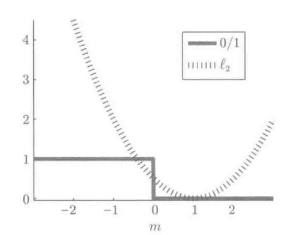间隔函数m=f(x)y的0/1损失和L2损失
上图表示的是m=f(x)y函数的0/1损失的例子。在0/1损失函数中，当m>0的时候，损失为0；当m≤0的时候，损失为1.0/1损失函数并不依赖与m值的大小，即使m是非常小的数值，只要是正数，损失就为零；而只要m是零以下的数值，损失就都为1.所以，m应该竟可能的取较大的数，这样学习的结果就会更加的稳定。

4.多类别的情况

前面介绍的都是两类别模式识别问题。然而在实际问题中，类别往往不仅仅只有两个，比如字母的手写识别需要26个类别，而汉字的识别更需要成百上千个类别。
多类别的模式识别问题的直接解决方案后面会专门开辟几个博文来讨论。这里将介绍两种利用2类别的模式识别算法解决多类别问题的方法。
4.1 一对多法用于多分类问题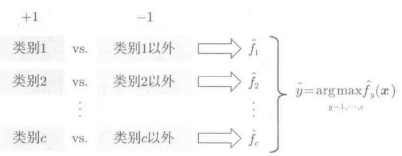使用一对多法进行分类

该方法首先解决2类别的分类问题。对于所有的与y=1,...,c相对应的类别，设其标签为+1，而对于剩余的y以外的所有类别，则设其标签为-1.在对样本x进行分类时，利用从各个2类别分类问题中得到的c个识别函数：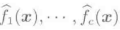对训练样本进行预测，并计算其函数值，期预测类别y'即为函数值最大时所对应的哪一类。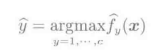在一对多方法中，从各个2类别的分类问题中训练得到c个识别函数f1(x),f2(x),...,fc(x)的输出，表示的是测试样本x属于类别y的概率，概率最大的哪一个就是测试样本x所属的类别。
4.2 一对一法用于多分类问题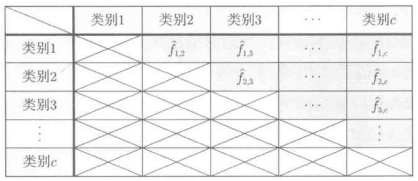在该方法中，首先对于所有的与y,y'=1,...,c相对应的类别，在任意两类之间训练一个分类器，属于类别y的标签设为+1，属于类别y'的标签设置为-1，通过这样的方式，利用2类别的分类算法进行求解。
在对样本x进行分类时，利用从各个2类别的分类问题中得到的c(c-1)/2个识别函数对训练样本进行预测，再用头皮案发决定其最终类别。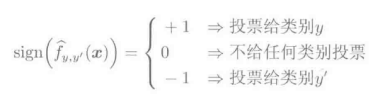得票数最多的类别就是样本x所属的类别。
4.2 讨论
在一对多方法中，对2类别问题进行了c次求解，而一对一方法则需要进行c(c-1)/2次求解。另一方面，在一对一方法中，对于每个2类别分类器，只需要2类的训练样本即可完成训练、学习。而在一对多方法中，对于每个2类别分类器，需要所有类别的训练样本都参与才能完成。
这里还要对比一下“直接法”和“间接法”。
虽然目前有多类问题的直接求解方法，但是并不是说对于多类问题直接进行求解一定是最好的选择。为什么这么说呢？因为与通用2类别分类问题间接求解相比，直接求解的计算一般更加的困难。因此，在实际应用中，更应该结合实际情况选择适应的方法。


展开全文• 决策树（Decision Tree）是一种基本的分类与回归方法。本文会讨论决策树中的分类树与回归树，后续文章会继续讨论决策树的Boosting和Bagging的相关方法。决策树由结点和有向边组成。结点有两种类型：内部结点和叶结点...
分类目录：《深入理解机器学习》总目录
相关文章：
基于决策树的模型（一）分类树和回归树
基于树的模型（二）：集成学习之Bagging和Random Forest
基于树的模型（三）：集成学习之GBDT和XGBoost
基于树的模型（四）：随机森林的延伸——深度森林（gcForest）
基于树的模型（五）：从零开始用Python实现ID3决策树
基于树的模型（六）：Python实现CART决策树并利用Tkinter构建GUI对决策树进行调优
基于树的模型（七）：RF/XGBoost等算法实践与决策树Scala实践等（材料准备中）

决策树（Decision Tree）是一种基本的分类与回归方法，当决策树用于分类时称为分类树，用于回归时称为回归树。本文主要讨论决策树中的分类树与回归树的一些基本理论，后续文章会继续讨论决策树的Boosting和Bagging相关方法。
决策树由结点和有向边组成。结点有两种类型：内部结点和叶结点，其中内部结点表示一个特征或属性，叶结点表示一个类。一般的，一棵决策树包含一个根结点、若干个内部结点和若干个叶结点。叶结点对应于决策结果，其他每个结点则对应于一个属性测试。每个结点包含的样本集合根据属性测试的结果被划分到子结点中，根结点包含样本全集，从根结点到每个叶结点的路径对应了一个判定测试序列。在下图中，圆和方框分别表示内部结点和叶结点。决策树学习的目的是为了产生一棵泛化能力强，即处理未见示例能力强的决策树。分类树
分类树是一种描述对实例进行分类的树形结构。在使用分类树进行分类时，从根结点开始，对实例的某一特征进行测试，根据测试结果，将实例分配到其子结点。这时，每一个子结点对应着该特征的一个取值。如此递归地对实例进行测试并分配，直至达到叶结点。最后将实例分到叶结点的类中。
假设给定训练数据集：
$D=\{(x_1, y_1), (x_2, y_2), ..., (x_N, y_N)\}$其中，$x_i=(x_i^{(1)}, x_i^{(2)}, ..., x_i^{(n)})^T,$为输入实例，即特征向量，$n$为特征个数，$i=1，2…，N$，$N$为样本容量，$y_i \in \{ 1, 2, ..., K\}$为类标。分类树学习的目标是根据给定的训练数据集构建一个决策树模型，使它能够对实例进行正确的分类。
决策树学习本质上是从训练数据集中归纳出一组分类规则。与训练数据集不相矛盾的决策树（即能对训练数据进行正确分类的决策树）可能有多个，也可能一个也没有。我们需要的是一个与训练数据矛盾较小的决策树，同时具有很好的泛化能力。从另一个角度看，决策树学习是由训练数据集估计条件概率模型。基于特征空间划分的类的条件概率模型有无穷多个，我们选择的条件概率模型应该不仅对训练数据有很好的拟合，而且对未知数据有很好的预测。
决策树学习用损失函数表示这一目标，其损失函数通常是正则化的极大似然函数，决策树学习的策略是以损失函数为目标函数的最小化。当损失函数确定以后，学习问题就变为在损失函数意义下选择最优决策树的问题。因为从所有可能的决策树中选取最优决策树是NP完全问题，所以现实中决策树学习算法通常采用启发式方法，近似求解这一最优化问题。这样得到的决策树是次最优的。

决策树分类算法
输入：
$\qquad$ 训练集：$D = {(x_1, y_1), (x_2, y_2), \cdots, (x_N, y_N)}$
$\qquad$ 属性集：$A = {a_1, a_2, \cdots, a_n}$
过程：
$\qquad$ 函数$TreeGenerate(D, A)$
输出：
$\qquad$ 以node为根节点的决策树
算法：
（ 1 ） 生成结点根node
（ 2 ） if $D$中样本全属于同一类别$C_k$ then
（ 3 ） $\quad$ 将node标记为$C_k$类叶结点
（ 4 ） $\quad$ return
（ 5 ） end if
（ 6 ） if $A = \varnothing$ OR $D$中样本在$A$上取值相同 then
（ 7 ） $\quad$ 将node标记为叶结点，其类别标记为$D$中样本数最多的类
（ 8 ） $\quad$ return
（ 9 ）end if
（10）从$A$中选择最优划分属性$a_*$
（11）for $a_*$ 的每一个值$a_*^v$ do
（12）$\quad$ 为node生成一个分支：令$D_v$表示$D$中在$a_*$上取值为$a_*^v$的样本子集
（13）$\quad$  if $D_v$为空  then
（14）$\qquad$ 将分支结点标记为叶结点，其类别标记为$D$中样本最多的类
（15）$\qquad$ return
（16）$\quad$  else
（17）$\qquad$ 以$TreeGenerate(D_v, A - \{a_*\})$为分支结点
（18）$\quad$ end if
（19）end for

决策树学习的算法通常是一个递归地选择最优特征，并根据该特征对训练数据进行分割，使得对各个子数据集有一个最好的分类的过程。这一过程对应着对特征空间的划分，也对应着决策树的构建。开始，构建根结点，将所有训练数据都放在根结点。选择一个最优特征，按照这一特征将训练数据集分割成子集，使得各个子集有一个在当前条件下最好的分类。如果这些子集已经能够被基本正确分类，那么构建叶结点，并将这些子集分到所对应的叶结点中去，如果还有子集不能被基本正确分类，那么就对这些子集选择新的最优特征，继续对其进行分割，构建相应的结点。如此递归地进行下去，直至所有训练数据子集被基本正确分类，或者没有合适的特征为止。最后每个子集都被分到叶结点上，即都有了明确的类。这就生成了一棵决策树。
从上述过程中就可以看出，决策树的生成是一个递归过程。在决策树基本算法中，有三种情形会导致递归返回

当前结点包含的样本全属于同一类别，无需划分
当前属性集为空，或是所有样本在所有属性上取值相同，无法划分
当前结点包含的样本集合为空，不能划分

在第二种情形下，我们把当前结点标记为叶结点，并将其类别设定为该结点所含样本最多的类别。在第三种情形下，同样把当前结点标记为叶结点，但将其类别设定为其父结点所含样本最多的类别。这两种情形的处理实质不同：第二种情况是在利用当前结点的后验分布，而第三种情况则是把父结点的样本分布作为当前结点的先验分布。
以上方法生成的决策树可能对训练数据有很好的分类能力，但对未知的测试数据却未必有很好的分类能力，即可能发生过拟合现象。我们需要对已生成的树自下而上进行剪枝，将树变得更简单，从而使它具有更好的泛化能力。具体地，就是去掉过于细分的叶结点，使其回退到父结点，甚至更高的结点，然后将父结点或更高的结点改为新的叶结点。如果特征数量很多，也可以在决策树学习开始的时候，对特征进行选择，只留下对训练数据有足够分类能力的特征。
可以看出，决策树学习算法包含特征选择、决策树的生成与决策树的剪枝过程。由于决策树表示一个条件概率分布，所以深浅不同的决策树对应着不同复杂度的概率模型。决策树的生成对应于模型的局部选择，决策树的剪枝对应于模型的全局选择。决策树的生成只考虑局部最优，相对地，决策树的剪枝则考虑全局最优。
决策树模型呈树形结构，在分类问题中，表示基于特征对实例进行分类的过程。它可以认为是if-then规则的集合，也可以认为是定义在特征空间与类空间上的条件概率分布。分类树具有良好的可读性与分类速度快的优点。分类树在学习时，利用训练数据，根据损失函数最小化的原则建立分类树模型，在预测时，对新的数据，利用分类树模型进行分类。决策树学习通常包括3个步骤：特征选择、决策树的生成和决策树的修剪。
决策树与if-then规则
可以将决策树看成一个if-then规则的集合：由决策树的根结点到叶结点的每一条路径构建一条规则，路径上内部结点的特征对应着规则的条件，而叶结点的类对应着规则的结论。决策树的路径或其对应的if-then规则集合具有一个重要的性质——互斥并且完备。这就是说，每一个实例都被一条路径或一条规则所覆盖，而且只被一条路径或一条规则所覆盖。这里所谓覆盖是指实例的特征与路径上的特征一致或实例满足规则的条件。
决策树与条件概率分布
决策树还表示给定特征条件下类的条件概率分布。这一条件概率分布定义在特征空间的一个划分上。将特征空间划分为互不相交的单元或区域，并在每个单元定义一个类的概率分布就构成了一个条件概率分布。决策树的一条路径对应于划分中的一个单元。决策树所表示的条件概率分布由各个单元给定条件下类的条件概率分布组成。假设$X$为表示特征的随机变量，$Y$为表示类的随机变量，那么这个条件概率分布可以表示为$P（Y|X）$。$X$取值于给定划分下单元的集合，$Y$取值于类的集合。各叶结点（单元）上的条件概率往往偏向某一个类，即属于某一类的概率较大。决策树分类时将该结点的实例强行分到条件概率大的那一类去。
决策树的优缺点

计算复杂度不高
对中间缺失值不敏感
解释性强，在解释性方面甚至比线性回归更强
与传统的回归和分类方法相比，决策树更接近人的决策模式
可以用图形表示，非专业人士也可以轻松理解
可以直接处理定性的预测变量而不需创建哑变量
决策树的预测准确性一般比回归和分类方法弱，但可以通过用集成学习方法组合大量决策树，显著提升树的预测效果

特征选择
特征选择在于选取对训练数据具有分类能力的特征。这样可以提高决策树学习的效率。如果利用一个特征进行分类的结果与随机分类的结果没有很大差别，则称这个特征是没有分类能力的。经验上扔掉这样的特征对决策树学习的精度影响不大。比如，我们希望构建一棵决策树来根据不同人的各种属性来预测每个人性别，那么对于属性“头发的长度”可能就要比属性“头发的颜色”所能包含的信息更多。因为一般来说，男生的头发要比女生的头发短，所以我们希望“头发的长度”这个属性处于决策树的上部。随着划分过程不断进行，我们希望决策树的分支结点所包含的样本尽可能属于同一类别，即结点的“纯度”（purity）越来越高。
信息增益
为了便于说明信息增益，先给出熵与条件熵的定义。在信息论与概率统计中，熵（entropy）是表示随机变量不确定性的度量。设$X$是一个取有限个值的离散随机变量，其概率分布为:
$P(X = x_i) = p_i, i = 1, 2, \cdots, n$
则随机变量$X$的熵定义为：
$H(X) = -\sum_{i = 1}^n p_i \log p_i$
在上式中，若$p_i = 0$，则定义$p_i \log p_i = 0$。通常，上式中的对数以$2$为底或以$e$为底（自然对数），这时熵的单位分别称作比特（bit）或纳特（nat）.由定义可知，熵只依赖于$X$的分布，而与$X$的取值无关，所以也可将$X$的熵记作$H(p)$，即:
$H(p) = -\sum_{i = 1}^n p_i \log p_i$
由此可见，熵越大，随机变量的不确定性就越大。从熵的定义可验证
$0 \leq H(p) \leq \log n$
当随机变量只取两个值，例如1，0时，即$X$的分布为:
$P(X = 1) = p,\quad P(X = 0) = 1-p, \quad 0≤p≤1$
其熵为：
$H(p) = -p \log_2 p - (1 - p)\log_2 (1 - p)$
这时，熵$H(p)$随概率$p$变化的曲线如下图所示（单位为比特）：当$p = 0$或$p = 1$时$H(p) = 0$，随机变量完全没有不确定，当$p = 0.5$时，$H(p) = 1$，熵取值最大，随机变量不确定性最大。
设有随机变量$(X, Y)$，其联合概率分布为：
$P(X = x_i, Y = y_i) = p_{ij} \quad \begin{cases} i = 1, 2, \cdots, n \\ j = 1, 2, \cdots, m \end{cases}$
条件熵$H(Y|X)$表示在已知随机变量$X$的条件下随机变量$Y$的不确定性。随机变量$X$给定的条件下随机变量$Y$的条件熵（conditional entropy）$H(Y|X)$，定义为$X$给定条件下$Y$的条件概率分布的熵对$X$的数学期望：
$H(Y|X) = \sum_{i = 1}^n p_iH(Y|X = x_i)$
其中，$p_i = P(X = x_i), i = 1, 2, \cdots, n$。
当熵和条件熵中的概率由数据估计（如极大似然估计）得到时，所对应的熵与条件熵分别称为经验熵（empirical entropy）和经验条件熵（empirical conditional entropy）。此时，如果有0概率，令$0\log0 = 0$。
信息增益（information gain）表示得知特征$X$的信息而使得类$Y$的信息的不确定性减少的程度。特征$a_*$对训练数据集$D$的信息增益$g(D, a_*)$，定义为集合$D$的经验熵$H(D)$与特征$a_*$给定条件下$D$的经验条件熵$H(D|a_*)$之差，即：
$g(D, a_*) = H(D) - H(D|a_*)$
一般地，熵$H(Y)$与条件熵$H(Y|X)$之差称为互信息（mutual information）。决策树学习中的信息增益等价于训练数据集中类与特征的互信息。
决策树学习应用信息增益准则选择特征。给定训练数据集$D$和特征$a_*$，经验熵$H(D)$表示对数据集$D$进行分类的不确定性。而经验条件熵$H(D|a_*)$表示在特征$a_*$给定的条件下对数据集$D$进行分类的不确定性。那么它们的差，即信息增益，就表示由于特征$a_*$而使得对数据集$D$的分类的不确定性减少的程度。显然，对于数据集$D$而言，信息增益依赖于特征，不同的特征往往具有不同的信息增益，信息增益大的特征具有更强的分类能力。
根据信息增益准则的特征选择方法：对训练数据集（或子集）$D$，计算其每个特征的信息增益，并比较它们的大小，选择信息增益最大的特征。
设训练数据集为$D$，$|D|$表示其样本容量，即样本个数。设有$K$个类$C_k$，$k=1, 2, \cdots, K$，$|C_k|$为属于类$C_k$的样本个数，$\sum_{k=1}^K |C_k| = |D|$。设特征$a_*$有$V$个不同的取值$\{ a_*^1, a_*^2, \cdots, a_*^V\}$，根据特征$a_*$的取值将$D$划分为$V$个子集$D_1, D_2, \cdots, D_V$，$|D_t|$为$D_t$的样本个数，$\sum_{i=1}^n|D_t|=|D|$。记子集$D_i$中属于类$C_k$的样本的集合为$D_{ik}$。即$D_{ik} = D_i \cap C_k$，$|D_{ik}|$为$D_{ik}$的样本个数。于是计算信息增益的方法如下：

信息增益
输入：训练数据集$D$和特征$a_*$
输出：特征$a_*$对训练数据集$D$的信息增益$g(D, a_*)$
1.计算数据集$D$的经验熵$H(D)$：$H(D) = -\sum_{k=1}^K \frac{C_k}{D}\log_2\frac{C_k}{D}$
2.计算特征$A$对数据集$D$的经验条件熵$H(D|A)$：$H(D|A) = \sum_{i=1}^n\frac{|D_i|}{D}H(D_i)=-\sum_{i=1}^n\frac{|D_i|}{D}\sum_{k=1}^K\frac{D_{ik}}{D_i}\log_2\frac{D_{ik}}{D_i}$
3.计算信息增益：$g(D, a_*) = H(D) - H(D|a_*)$

一般而言，信息增益越大，则意味着使用特征$a_*$来进行划分所获得的“纯度提升”越大。因此，我们可用信息增益来进行决策树的划分属性选择，即在上述决策树分类算法第10行使用$a_* = \text{arg}\ \max_{a \in A}g(D, a)$选择最优划分属性。著名的ID3决策树学习算法就是以信息增益为准则来选择划分属性。
ID3算法的核心是在决策树各个结点上应用信息增益准则选择特征，递归地构建决策树。具体方法是：从根结点开始，对结点计算所有可能的特征的信息增益，选择信息增益最大的特征作为结点的特征，由该特征的不同取值建立子结点。之后，再对子结点递归地调用以上方法，构建决策树，直到所有特征的信息增益均很小或没有特征可以选择为止，最终得到一个决策树。ID3相当于用极大似然法进行概率模型的选择。

ID3算法
输入：训练数据集$D$，特征集$A$，阈值$\epsilon$
输出：决策树$T$
1.若$D$中所有实例属于同一类$C_k$，则$T$为单结点树，并将类$C_k$作为该结点的类标记，返回决策树$T$
2.若$A = \varnothing$，则$T$为单结点树，并将$D$中实例数最大的类$C_k$，作为该结点的类标记，返回决策树$T$
3.否则，计算$A$中各特征对$D$的信息增益，选择信息增益最大的特征$a_*$
4.如果$a_*$的信息增益小于阈值$\epsilon$，则置$T$为单结点树，并将$D$中实例数最大的类$C_k$作为该结点的类标记，返回决策树$T$
5.否则，对$a_*$的每一可能值$a_*^v$，依$a_* = a_*^v$将$D$分割为若干非空子集$D_v$，将$D_v$中实例数最大的类作为标记，构建子结点，由结点及其子结点构成决策树$T$，返回决策树$T$
6.对第$v$个子结点，以$D_v$为训练集，以$A - \{a_*\}$为特征集，递归地调用第(1)步~第(5)步，得到子树$T_v$，并返回子树$T_v$

信息增益率
信息增益值的大小是相对于训练数据集而言的，并没有绝对意义。在训练数据集的经验熵大的时候，信息增益值会偏大。反之，信息增益值会偏小。使用信息增益率（information gain ratio）可以对这一问题进行校正。这是特征选择的另一准则。特征$a_*$对训练数据集$D$的信息增益率$g_g(D, a_*)$定义为其信息增益$g(D, a_*)$与训练数据集$D$的经验熵$H(D)$之比：
$g_g(D, a_*) = \frac{g(D, a_*)}{H(D)}$
如前文所说，信息增益准则对可取值数目较多的属性有所偏好，为减少这种偏好可能带来的不利影响，著名的C4.5决策树算法不直接使用信息增益来选择划分属性，而是使用信息增益率来选择最优划分属性。

C4.5算法
输入：训练数据集$D$，特征集$A$，信息增益率阈值$\epsilon$，信息增益阈值$\alpha$
输出：决策树$T$
1.若$D$中所有实例属于同一类$C_k$，则$T$为单结点树，并将类$C_k$作为该结点的类标记，返回决策树$T$
2.若$A = \varnothing$，则$T$为单结点树，并将$D$中实例数最大的类$C_k$，作为该结点的类标记，返回决策树$T$
3.否则，计算$A$中各特征对$D$的信息增益和信息增益率，在信息增益大于$\alpha$的特征中选择信息增益率最大的特征$a_*$
4.如果$a_*$的信息增益率小于阈值$\epsilon$，则置$T$为单结点树，并将$D$中实例数最大的类$C_k$作为该结点的类标记，返回决策树$T$
5.否则，对$a_*$的每一可能值$a_*^v$，依$a_* = a_*^v$将$D$分割为若干非空子集$D_v$，将$D_v$中实例数最大的类作为标记，构建子结点，由结点及其子结点构成决策树$T$，返回决策树$T$
6.对第$v$个子结点，以$D_v$为训练集，以$A - \{a_*\}$为特征集，递归地调用第(1)步~第(5)步，得到子树$T_v$，并返回子树$T_v$

需注意的是，信息增益率准则对可取值数目较少的属性有所偏好，因此，C4.5算法并不是直接选择增益率最大的候选划分属性，而是使用了一个启发式的方法选择最优划分属性：先从候选划分属性中找出信息增益高于平均水平的属性，再从中选择增益率最高的.。
连续值处理
实际的任务中常会遇到连续属性，对于全部为连续属性的样本来说，我们一般使用回归决策树来处理。C4.5算法则采用了二分法对连续属性进行处理。由于连续属性的可取值数目不再有限，因此，不能直接根据连续属性的可取值来对结点进行划分。此时，连续属性离散化技术可派上用场。最简单的策略是采用二分法对连续属性进行处理。
给定样本集$D$和连续属性$a$，假定$a$在$D$上出现了$n$个不同的取值，将这些值从小到大进行排序，记为$\{a_1, a_2, a_3, \cdots, a_n\}$。基于划分点$t$可将$D$分为子集$D_t^+$和$D_t^-$，其中$D_t^+$包含那些在属性$a$上取值大于$t$的样本，而$D_t^-$则包含那些在属性$a$上取值不大于$t$的样本。显然，对相邻的属性取值$a^i$与$a^{i + 1}$来说，$t$在区间$[a^i, a^{i + 1})$中取任意值所产生的划分结果相同.因此，对连续属性$a$，我们可考察包含$n-1$个元素的候选划分点集合：
$T_a = \{\frac{a^i + a^{i + 1}}{2} \ | \ 1 \leq i \leq n - 1\}$
即把区间$[a^i, a^{i + 1})$的中位点$\frac{a^i + a^{i + 1}}{2}$作为候选划分点。然后，我们就可像离散属性值一样来考察这些划分点，选取最优的划分点进行样本集合的划分：
\begin{aligned} Gain(D, a) &= \max_{t \in T_a} Gain(D, a, t)\\ & = \max_{t \in T_a} Ent(D) - \sum_{\lambda \in \{-, +\}} \frac{D^\lambda _t}{D}Ent(D^\lambda _t) \end{aligned}
其中$Gain(D, a, t)$是样本集$D$基于划分点$t$二分后的信息增益。于是，我们就可选择使$Gain(D, a, t)$最大化的划分点。
缺失值处理
现实任务中常会遇到不完整样本，即样本的某些属性值缺失。且在属性数目较多的情况下，有时会有大量样本出现缺失值。如果简单地放弃不完整样本，仅使用无缺失值的样本来进行学习，显然是对数据信息极大的浪费。显然，有必要考虑利用有缺失属性值的训练样例来进行学习。
划分属性的选择
给定训练集$D$和属性$a$，令$\tilde{D}$表示$D$中在属性$a$上没有缺失值的样本子集。显然，我们仅可根据$\tilde{D}$来判断属性$a$的优劣。假定属性$a$有$V$个可取值$\{a^1, a^2, a^3, \cdots, a^V\}$，令$\tilde{D}^v$表示$\tilde{D}$中在属性$a$上取值为$a^v$的样本子集，$\tilde{D}_k$表示$\tilde{D}$中属于第$k$类$(k = 1, 2, 3, \cdots, K)$的样本子集，则显然有$\tilde{D} = \bigcup^K_{k = 1}\tilde{D}_k$与$\tilde{D} = \bigcup^V_{v = 1}\tilde{D}^v$。假定我们为每个样本$x$赋予一个权重$\omega_x$，并定义：
\begin{aligned} \rho & = \frac{\sum_{x \in \tilde{D}}\omega_x}{\sum_{x \in D}\omega_x}\\ \tilde{p}_k & = \frac{\sum_{x \in \tilde{D}_k}\omega_x}{\sum_{x \in \tilde{D}}\omega_x}\\ \tilde{r}_v & = \frac{\sum_{x \in \tilde{D}^v}\omega_x}{\sum_{x \in \tilde{D}}\omega_x} \end{aligned}
直观地看，对于属性$a$，$\rho$表示无缺失值样本所占的比例，$\tilde{p}_k$表示无缺失值样本中第$k$类所占的比例，$\tilde{r}_v$则表示无缺失值样本中在属性$a$上取值$a^v$的样本所占的比例。基于上述定义，我们可将信息增益的计算式推广为：
\begin{aligned} Gain(D, a) &= \rho \times Gain(\tilde{D}, a)\\ & = \rho \times (Ent(\tilde{D}) - \sum_{x = 1}^V \tilde{r}_v Ent(\tilde{D}^v)) \end{aligned}
对样本进行划分
根据上面的定义，若样本$x$在划分属性$a$上的取值已知，则将$x$划入与其取值对应的子结点，且样本权值在子结点中保持为$\omega_x$。若样本$x$在划分属性$a$上的取值未知，则将$x$同时划入所有子结点，且样本权值在与属性值$a^v$对应的子结点中调整为$\tilde{r}_v \times \omega_x$。直观地看，这就是让同一个样本以不同的概率划入到不同的子结点中去。
基尼指数
数据集$D$的纯度还可用基尼值来度量：
$Gini(D) = \sum^K_{k=1}p_k(1 - p_k) = 1 - \sum^K_{k=1}p_k^2$
其中，$K$为类别数，$p_k$为样本点属于第$k$类的概率。对于二类分类问题，若样本点属于第1个类的概率是$p$，则概率分布的基尼指数为：
$Gini(D) = 2p(1 - p)$
直观来说，$Gini(D)$反映了从数据集$D$中随机抽取两个样本，其类别标记不一致的概率。因此，$Gini(D)$越小，则数据集$D$的纯度越高。对于属性$a$的基尼指数定义为:
$Gini\_index(D, a) = \sum^V_{v = 1}\frac{D^v}{D}Gini(D^v)$
在CART算法中，我们在候选属性集合$A$中，选择那个使得划分后基尼指数最小的属性作为最优划分属性，即：$a_* = \text{arg}\min_{a \in A}Gini\_index(D, a)$
在二类分类问题中，基尼指数$Gini(D)$、熵$H(p)$的一半，和分类误差率的关系：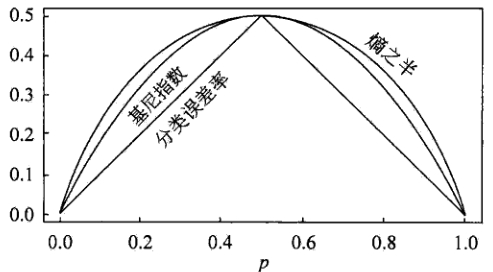其中，横坐标表示概率$p$，纵坐标表示损失。可以看出基尼指数和熵的一半的曲线很接近，都可以近似地代表分类误差率。
分类树的剪枝
剪枝（pruning）是决策树学习算法对付“过拟合”的主要手段。在决策树学习中，为了尽可能正确分类训练样本，结点划分过程将不断重复，有时会造成决策树分支过多，这时就可能因为对训练样本学习得“太好”了，以致于把训练集自身的一些特点当作所有数据都具有的一般性质而导致过拟合。因此，可通过主动去掉一些分支来降低过拟合的风险。决策树剪枝的基本策略有预剪枝和后剪枝。
预剪枝
预剪枝是指在决策树生成过程中，对每个结点在划分前先进行估计，若当前结点的划分不能带来决策树泛化性能提升，则停止划分并将当前结点标记为叶结点。停止决策树生长常用方法：

定义一个高度，当决策树达到该高度时就停止决策树的生长
达到某个节点的实例具有相同的特征向量，即使这些实例不属于同一类，也可以停止决策树的生长。这个方法对于处理数据的数据冲突问题比较有效。
定义一个阈值，当达到某个节点的实例个数小于阈值时就可以停止决策树的生长
定义一个阈值，通过计算每次扩张对系统性能的增益，并比较增益值与该阈值大小来决定是否停止决策树的生长。

后剪枝
后剪枝则是先从训练集生成一棵完整的决策树，然后自底向上地对非叶结点进行考察，若将该结点对应的子树替换为叶结点能带来决策树泛化性能提升，则将该子树替换为叶结点。相比于预剪枝，后剪枝更常用，因为在预剪枝中精确地估计何时停止树增长很困难。
错误率降低剪枝（REP，Reduced-Error Pruning）
错误率降低剪枝方法是一种比较简单的后剪枝的方法。在该方法中，可用的数据被分成两个样例集合：首先是训练集，它被用来形成学习到的决策树，另一个是与训练集分离的验证集，它被用来评估这个决策树在后续数据上的精度，确切地说是用来评估修剪这个决策树的影响。学习器可能会被训练集中的随机错误和巧合规律所误导，但验证集合不大可能表现出同样的随机波动。所以验证集可以用来对过度拟合训练集中的虚假特征提供防护检验。
错误率降低剪枝方法考将树上的每个节点作为修剪的候选对象，再决定是对该节点进行剪枝：

删除以此结点为根的子树
使其成为叶子结点
当修剪后的树对于验证集合的性能不比修剪前的树的性能差时，则确认删除该结点，否则恢复该节点

因为训练集合的过拟合，使得验证集合数据能够对其进行修正，反复进行上面的操作，从底向上的处理结点，删除那些能够提高验证集合的精度的结点，直到进一步修剪会降低验证集合的精度为止。
错误率降低剪枝方法是最简单的后剪枝方法之一，不过由于使用独立的测试集，原始决策树相比，修改后的决策树可能偏向于过度修剪。这是因为一些不会再次在测试集中出现的很稀少的训练集实例所对应的分枝在剪枝过程中往往会被剪枝。尽管错误率降低剪枝方法有这个缺点，不过错误率降低剪枝方法仍然作为一种基准来评价其它剪枝算法的性能。它对于两阶段决策树学习方法的优点和缺点提供了一个很好的学习思路。由于验证集合没有参与决策树的构建，所以用错误率降低剪枝方法剪枝后的决策树对于测试样例的偏差要好很多，能够解决一定程度的过拟合问题。
悲观错误剪枝（PEP，Pesimistic-Error Pruning）
悲观错误剪枝方法是根据剪枝前后的错误率来判定子树的修剪。它不需要像错误率降低修剪方法那样，需要使用部分样本作为测试数据，而是完全使用训练数据来生成决策树，并进行剪枝，即决策树生成和剪枝都使用训练集。
该方法引入了统计学中连续修正的概念弥补错误率降低剪枝方法中的缺陷，在评价子树的训练错误公式中添加了一个常数，即假定每个叶子结点都自动对实例的某个部分进行错误的分类。
把一颗具有多个叶子节点的子树的分类用一个叶子节点来替代的话，在训练集上的误判率肯定是上升的，但是在新数据上不一定。于是我们把子树的误判计算加上一个经验性的惩罚因子来做是否剪枝的考量指标。对于一个叶子节点，它覆盖了$N$个样本，其中有$E$个错误，那么该叶子节点的错误率为$\frac{E + 0.5}{N}$。这个$0.5$就是惩罚因子，那么一颗子树，它有$L$个叶子节点，那么该子树的误判率估计为：
$\frac{\sum E_i +0.5 * L}{\sum N_i}$
这样的话，我们可以看到一棵子树虽然具有多个子节点，但由于加上了惩罚因子，所以子树的误判率计算未必占到便宜。剪枝后内部节点变成了叶子节点，其误判个数$E$也需要加上一个惩罚因子，变成$E+0.5$，那么子树是否可以被剪枝就取决于剪枝后的错误$E+0.5$在的标准误差内。对于样本的误差率$e$，我们可以根据经验把它估计成各种各样的分布模型，比如二项式分布、正态分布等。如果$E+0.5 < E_i + SE(E_i)$则对$i$进行剪枝。
代价复杂度剪枝（CCP，Cost-Complexity Pruning)
代价复杂度剪枝算法为子树$T_t$定义了代价和复杂度，以及一个可由用户设置的衡量代价与复杂度之间关系的参数$α$。其中，代价指在剪枝过程中因子树$T_t$被叶节点替代而增加的错分样本，复杂度表示剪枝后子树$T_t$减少的叶结点数，$α$则表示剪枝后树的复杂度降低程度与代价间的关系，定义为：
$\alpha = \frac{R(t) - R(T_t)}{|N_t| - 1}$
其中，$|N_t|$是子树$T_t$中的叶节点数，$R(t) = r(t) * p(t)$为结点$t$的错误代价，$r(t)$为结点$t$的错分样本率，$p(t)$为落入结点$t$的样本占所有样本的比例，$R(T_t) = \sum R(i)$是子树$T_t$错误代价，$i$为子树$T_t$的叶节点。

对于完全决策树$T$的每个非叶结点计算$α$值，循环剪掉具有最小$α$值的子树，直到剩下根节点，得到一系列的剪枝树$\{ T_0, T_1, T_2, \cdots, T_m \}$，其中$T_0$为原有的完全决策树，$T_m$为根结点，$T_{i +1}$为对$T_i$进行剪枝的结果
从子树序列中，根据真实的误差估计选择最佳决策树

REP
PEP
CCP

剪枝方式
自底向上
自顶向下
自底向上

计算复杂度
$O(n)$
$O(n)$
$O(n^2)$

误差估计
测试集上误差估计
使用连续纠正
标准误差

回归树
建立回归树的过程大致可以分为两步：

将预测变量空间（$X_1, X_2, X_3, \cdots, X_p$）的可能取值构成的集合分割成$J$个互不重叠的区域$\{ R_1, R_2, R_3, \cdots, R_J\}$
对落入区域$R_j$的每个观测值作同样的预测，预测值等于$R_j$上训练集的各个样本取值的算术平均数。

比如，在第一步中得到两个区域$R_1$和$R_2$，$R_1$中训练集的各个样本取值的算术平均数为10，$R_2$中训练集的各个样本取值的算术平均数为20。则对给定的观测值$X = x$，若$x \in R_1$，给出的预测值为10，若$x \in R_2$，则预测值为20。
所以，类似于上述决策树分类算法的第(10)步，关键在于如何构建区域划分$\{ R_1, R_2, R_3, \cdots, R_J\}$。事实上，区域的形状是可以为任意形状的，但出于模型简化和增强可解释性的考虑，这里将预测变量空间划分成高维矩形，我们称这些区域为称盒子。划分区域的目标是找到使模型的残差平方和$RSS$最小的矩形区域$\{ R_1, R_2, R_3, \cdots, R_J\}$。$RSS$的定义为：
$RSS = \sum^J_{j=1} \sum_{i \in R_j}(y_i - \hat{y}_{R_j})^2$
其中，$\hat{y}_{R_j}$是第$j$个矩形区域中训练集中各个样本取值的算术平均数。但是，要想考虑将特征空间划分为$J$个矩形区域的所有可能性，在计算上是不可行的。因此一般采用一种自上而下的贪婪法：递归二又分裂。“自上而下”指的是它从树顶端开始依次分裂预测变量空间，每个分裂点都产生两个新的分支。“贪婪”意指在建立树的每一步中，最优分裂确定仅限于某一步进程，而不是针对全局去选择那些能够在未来进程中构建出更好的树的分裂点。
在执行递归二又分裂时，先选择预测变量$X_j$和分割点$s$，将预测变量空间分为两个区域$\{ X|X_j 和$\{ X|X_j \geq s \}$，使$RSS$尽可能地减小。也就是说，考虑所有预测变量$X_1, X_2, X_3, \cdots, X_p$和与每个预测变量对应的$s$的取值，然后选择预测变量和分割点，使构造出的树具有最小的$RSS$。更详细地，对$j$和$s$，定义一对半平面：
$R_1(j, s) = \{ X|X_j
寻找$j$和$s$，使得下式最小：
$\sum_{x_i \in R_1(j, s)}(y_i - \hat{y}_{R_1})^2 + \sum_{x_i \in R_2(j, s)}(y_i - \hat{y}_{R_2})^2$
重复上述步骤，寻找继续分割数据集的最优预测变量和最优分割点，使随之产生的区域中的$RSS$达到最小。此时被分割的不再是整个预测变量空间，而是之前确定的两个区域之一。如此一来就能得到3个区域。接着进一步分割3个区域之一以最小化$RSS$。这一过程不断持续，直到符合某个停止准则，如我们在分类决策树中讨论到的前剪枝中的停止准则。
区域$\{ R_1, R_2, R_3, \cdots, R_J\}$产生后，就可以确定某一给定的测试数据所属的区域，并用这一区域训练集的各个样本取值的算术平均数作为与测试进行预测。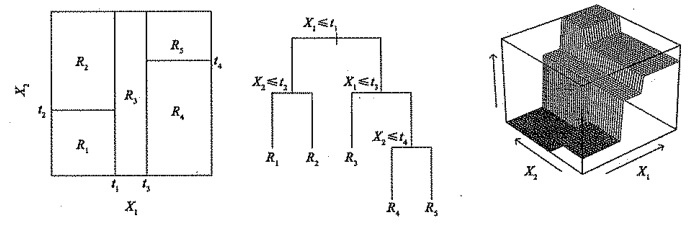回归树的剪枝
上述方法生成的回归树会在训练集中取得良好的预测效果，却很有可能造成数据的过拟合，导致在测试集上效果不佳。原因在于这种方法产生的树可能过于复杂。一棵分裂点更少、规模更小（区域$\{ R_1, R_2, R_3, \cdots, R_J\}$的个数更少）的树会有更小的方差和更好的可解释性（以增加微小偏差为代价）。针对上述问题，一种可能的解决办法是：仅当分裂使残差平方和$RSS$的减小量超过某阈值时，才分裂树结点。这种策略能生成较小的树，但可能产生过于短视的问题，一些起初看来不值得的分裂却可能之后产生非常好的分裂。也就是说在下一步中，$RSS$会大幅减小。
因此，更好的策略是生成一棵很大的树$T_0$，然后通过后剪枝得到子树。直观上看，剪枝的目的是选出使测试集预测误差最小的子树。子树的测试误差可以通过交叉验证或验证集来估计。但由于可能的子树数量极其庞大，对每一棵子树都用交叉验证来估计误差太过复杂。因此需要从所有可能的子树中选出一小部分再进行考虑。在回归树中，我们一般使用代价复杂度剪枝（CCP，Cost-Complexity Pruning），也称最弱联系剪枝（Weakest Link Pruning）。这种方法不是考虑每一棵可能的子树，而是考虑以非负调整参数$\alpha$标记的一系列子树。每一个$\alpha$的取值对应一棵子树$T \in T_0$，当$\alpha$一定时，其对应的子树使下式最小：
$\sum_{m=1}^{|T|} \sum_{x_i \in R_m}(y_i - \hat{y}_{R_m})^2 + \alpha |T|$
这里的$|T|$表示树$T$的结点数，$R_m$是第$m$个终端结点对应的矩形（预测向量空间的一个子集），$\hat{y}_{R_m}$是与$R_m$对应的预测值，也就是$R_m$中训练集的平均值。调整系数$\alpha$在子树的复杂性和与训练数据的契合度之间控制权衡。当$\alpha = 0$时，子树$T$等于原树$T_0$，因为此时上式只衡量了训练误差。而当$\alpha$增大时，终端结点数多的树将为它的复杂付出代价，所以使上式取到最小值的子树会变得更小。
当$\alpha$从0开始逐渐增加时，树枝以一种嵌套的、可预测的模式被修剪，因此获得与$\alpha$对应的所有子树序列是很容易的。可以用交又验证或验证集确定$\alpha$，然后在整个数据集中找到与之对应的子树：

回归决策树算法
1.利用递归二叉分裂在训练集中生成一额大树，只有当终端结点包含的观测值个数低于某个最小值时才停止。
2.对大树进行代价复杂性剪枝，得到一系列最优子树，子树是$\alpha$的函数。
3.利用$K$折交叉验诞选择$\alpha$。具体做法是将训练集分为$K$折。对所有$k=1, 2, 3, \cdots, K$，对训练集上所有不属于第$k$折的数据重复第(1)步~第(2)步得到与$\alpha$对应的子树，并求出上述子树在第$k$折上的均方预测误差。
4.每个$\alpha$会有相应的$K$个均方预测误差，对这$K$个值求平均，选出使平均误差最小的$\alpha$。
5.找出选定的$\alpha$在第(2)步中对应的子树。

决策树的基础即是上文所述的分类决策树与回归决策树，其预测准确性一般比回归和分类方法弱，但可以通过用集成学习方法组合大量决策树，显著提升树的预测效果，这些方法我将会在接下来的文章中继续阐述，欢迎关注学习讨论！


展开全文机器学习 决策树 回归树
• ## 最大似然分类法

万次阅读 多人点赞 2014-02-16 21:06:20
今天要介绍的是另外一种分类方法——最大似然分类法，它也是分类里面用得很多的一种分类方法，它在分类的时候，不仅考虑了待分类样本到已知类别中心的距离，而且还考虑了已知类别的分布特征，所以其分类
                                          最大似然分类法介绍
作者：liangdas
出处：简单点儿，通俗点儿，机器学习  http://blog.csdn.net/liangdas/article/details/19294951
前言：
最小距离分类法只考虑了待分类样本到各个类别中心的距离，而没有考虑已知样本的分布，所以它的分类速度快，但精度不高。今天要介绍的是另外一种分类方法——最大似然分类法，它也是分类里面用得很多的一种分类方法，它在分类的时候，不仅考虑了待分类样本到已知类别中心的距离，而且还考虑了已知类别的分布特征，所以其分类精度比最小距离分类法要高。

下面有一个图，黄色所示类别A的分布，蓝色是类别B的分布，类别A和B的类别中心都红色的点表示出来了，那么有一个未知类别的样本p，假设p到类别A的距离是Ap，到类别B的距离是Bp，现在Ap=Bp，那么如果用最小距离分类法，我们是不能判断样本p到底是属于A类还是B类的，所以这就是最小距离分类法的局限性。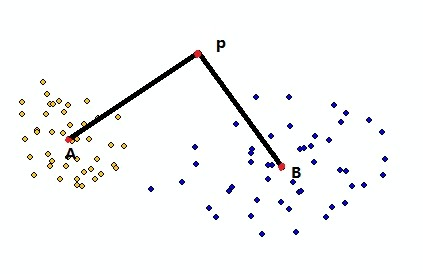感性认识：
假设在我们读书的时候，在一个月内，30天，你发现晚上11点以后实验室还亮着灯，你很好奇，每次推门进去，发现这30天内，实验室中不是甲就是乙，或者他们同时都在，你做了统计，是甲的天数是28天，是乙的次数是4天，然后有一天晚上11点，你发现实验室的灯又亮了，你同学问你：“你猜现在是谁在实验室呀？”，你会回答“应该是甲吧”。这其实就是生活中我们利用最大似然来分类的一个例子。
另外一个例子：现在我们有两个盒子：甲和乙，每个里面都装了100个球，其中甲中装了95个红球，5个黑球，乙中装了60个红球，40个黑球，现在有人从盒子里面取出了一个球，发现是红球，然后让你猜：“他是从哪个盒子里面取出来的？”，想必你会猜“甲”吧。
好了，其实我们生活中，已经对最大似然估计有了自己的经验体会，是不是还没有意识到它就是最大似然分类法呢？呵呵。
基本原理：
假设有两个事件A，B，我们通过先验的知识，知道A发生的条件下，x也发生的概率是P(x|A)； B发生的条件下，x也发生的概率是P(X|B)，那么，现在有一个事件x发生了，我们能否判断这个事件x是在A条件下，还是在B条件下发生的可能性大些呢？也就是要求出P（A|x）和P（B|x）哪一个最大？对分类问题而言，哪一个概率大，我们就说x属于哪一类。
我们回忆下贝叶斯公式：P(A|B)=P(B|A)*P(A)/P(B)
从贝叶斯公式中我们可以看到，求概率P(A|B)的问题转化成了求P(B|A)，P(A)和P(B)的问题，其实里面真正的精华是：概率P(A|B)和P(B|A)的转换。因为通常，我们事先能知道P(A)，P(B)；或者是P(A)和P(B)在分类问题中是公共的项，可以约去；再或是他们的差异可以忽略不计，所以，要P(A|B)最大，也就是要P(B|A)最大！而对于P(B|A)，我们可以从事先已经发生的事件中，通过统计等数学方法计算得到，这样这个分类问题就好解了！我们可以提炼下面的目标函数：

其中是目标函数，是已知x事件发生了，那么它属于的概率；是类别对应的x的概率值；是类别发生的概率，是事件x发生的概率。
贝叶斯公式的经典之处就是将后验概率的问题转化成了先验概率的问题！
再回到上面的盒子和白球的问题中，A和B分别代表盒子甲和乙，事件x就是红球发生的概率，我们先验已经知道P(x|A）= 95/100=0.95，P(x|B)=60/100=0.6，我们要求的是P(A|x)和P(B|x)，然后比较哪个最大？
P(A|x)= P(A)*P(x|A)/P(x)
P(B|x)= P(B)*P(x|B)/P(x)
对于P(A), P(B)，因为我们目前总共只有两个盒子甲和乙，也就是A和B，它们各有一个，所以P(A)=P(B)=1/2=0.5。
对于P(x)，因为P(A|x)和P(B|x）的贝叶斯表达式中都有P(x)这一项，所以，P（x）作为公共项，我们可以不考虑。如果硬是想知道它的值，那么因为是随机取一个球，P(x）=(95+60)/(100+100)。
这样我们就可以轻松的知道P(A|x)大，还是P(B|x)大了。
最大似然分类又叫贝叶斯分类。
N维空间的最大似然分类法原理：
上面的例子中， x都是二项分布的，也就是x的取值只取两个值，通常为0，1，发生或者不发生等，但是在实际问题中，x往往不是服从这么一个简单的二项分布，而很有可能是其他更复杂一些的分布。
在统计学和模式识别中，对某一个待研究的集合，在经验的基础上，我们通常假设它是一个服从正太分布的变量集合，如一群人的身高、某各班级某一科目的考试成绩、某个类别的某一维特征，我们都假定它是服从正态分布的，也叫高斯分布，即用均值，和方差来描述的一组样本分布。对一维正态分布，它的概率密度函数表达式是：，表示的是变量在分布中的出现的概率。
通常，为了描述某一个对象，我们会从这个对象抽象出多个属性，每一个属性代表一个一维空间，多个属性就组成一个多维空间。对于多维空间的中的变量，它也有其正态分布的概率密度函数：

其中，是概率密度，是特征维数，又叫特征空间维数（对遥感图像分类来说， 就是波段的个数）；是n维空间中的一个向量；也是一个n向量，它是由每一维特征的均值组成的一个向量；是n维特征向量之间的协方差矩阵。
上面已经指出了，最大似然分类的目标函数是：

当x是一个多维空间中的一个向量，而且每一维空间都的分布都服从正态分布的时候，则x的概率密度函数是：

通常可以根据已知条件计算得到，或者其区别可以忽略不计。
是公共项，所以不用考虑其具体的值。
N维空间的最大似然分类法的化简：
到目前为止，我们利用上面的就可以计算出最大似然分类的结果了，但是在上面的计算公式中有一个指数的计算，我们知道指数计算在计算机中是特别耗费时间，当特征维数较多，待分类的类别个数也比较多的的时候，指数计算势必会导致最后的分类的速度变得很慢，所以能不能把指数计算去掉呢？答案当然是可以的，我们只需要对表达式取对数。
因为是公共项，所以，我们去掉，目标函数就变为：

对目标函数取对数：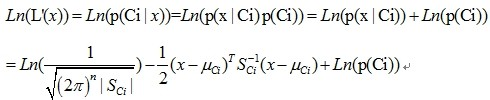在分类之前，我们为了消除量纲的影响，都需要对所有维的特征做归一化处理，使其服从标准正态分布，另外，如果我们在取特征的时候，每一维特征都取得非常好，它们之间相互独立（其实可以对特征做变换，使各维特征能够相互独立，如PCA，LDA等），如果上述条件都满足，每个特征都会具有相同的方差，不同维之间的协方差为0（因为不同维特征不相关），这种情况下，协方差矩阵是对角阵，且是一个单位阵
E，因此，每一类的协方差都相等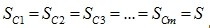，因此，公式化简为：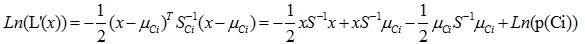如果各类的先验概率相等，即 的大小与类别无关，那么上面的式子中的最后一项又可以去掉，最终公式变成了：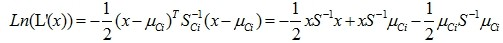到此为止，这个目标函数就已经没有了指数和对数计算了！就剩下一些矩阵的运算了，所以运算速度也得到了很大的提高！
在实际的使用当中，我们需要根据不同的问题特点，来确定最终能化简的程度。
参考资料：
百度文库——最大似然分类器的基本思想和数学原理：
http://wenku.baidu.com/view/12a5de46a8956bec0975e364.html
百度文库——最大似然分类算法原理及实现：
http://wenku.baidu.com/view/03a40da0f524ccbff12184c7.html
百度文库——矩阵模板类：
矩阵加减求逆运算的模板类:

ps：使用或者转载请标明出处，禁止以商业为目的的使用。
如果有需要word版，或者是pdf版的，请与我联系，QQ：358536026

`
展开全文算法
• ## 文本分类——常见分类模型

万次阅读 多人点赞 2018-11-06 17:37:56
文本分类方法模型主要分为两个大类，一类是基于规则的分类模型；另一类是基于概率统计的模型。 基于规则的模型   基于规则的分类模型相对简单，易于实现。它在特定领域的分类往往能够取得较好的效果。相对于...自然语言处理 机器学习
• ## 最大似然分类法MLC

万次阅读 2017-02-14 15:41:30
今天要介绍的是另外一种分类方法——最大似然分类法，它也是分类里面用得很多的一种分类方法，它在分类的时候，不仅考虑了待分类样本到已知类别中心的距离，而且还考虑了已知类别的分布特征，所以其分类精度比最小...算法
• 对于二分类问题，可将样例根据真实类别和分类器预测类别划分为： 真正例（True Positive，TP）：真实类别为正例，预测类别为正例。 假正例（False Positive，FP）：真实类别为负例，预测类别为正例。 假负例...
• 基于规则的分类是一种比较简单的分类技术，下面从以下几个方面对进行介绍  1.任务  所有的分类技术的任务都是利用数据集训练出分类器，然后为每条记录贴上标签，对进行分类，基于规则的分类任务也是如此。 ...
• ## 图像分类

万次阅读 多人点赞 2018-01-21 15:31:47
图像物体分类与检测算法综述 转自《计算机学报》 目录 图像物体分类与检测算法综述 目录 图像物体分类与检测概述 物体分类与检测的难点与挑战 物体分类与检测数据库 物体分类与检测发展历程 ...计算机视觉
• ## 分类器

万次阅读 多人点赞 2018-09-21 21:23:10
分类器的作用：常规任务是利用给定的类别、已知的训练数据来学习分类规则和分类器，然后对未知数据进行分类（或预测）。逻辑回归（logistics）、SVM等常用于解决二分类问题，对于多分类问题（multi-class ...
• ## Java异常分类

万次阅读 多人点赞 2012-11-27 15:46:48
} } 我们比较熟悉的Checked异常有 Java.lang.ClassNotFoundException Java.lang.NoSuchMetodException java.io.IOException 2.2 RuntimeException Runtime如除数是0和数组下标越界等，产生频繁，处理麻烦，若...
• 1 三分类 （1）说明简介 将白细胞分成三大类，小细胞群（淋巴细胞）、中间细胞群（嗜酸细胞、嗜碱细胞及单核细胞）、大细胞群（中性粒细胞）。 （2）检测方法 基于库尔特计数原理，以细胞体积的大小进行计数和...
• 详解sigmoid与softmax， 多分类及多标签分类激活函数介绍sigmoid激活函数sigmoid激活函数的性质sigmoid激活函数的使用 激活函数介绍 对于熟悉机器学习或神经网络的读者来说，sigmoid与softmax两个激活函数并不陌生，...softmax
• ## 机器学习之KNN最邻近分类算法

万次阅读 多人点赞 2018-09-15 13:13:33
KNN（K-Nearest Neighbor）最邻近分类算法是数据挖掘分类（classification）技术中最简单的算法之一，指导思想是”近朱者赤，近墨者黑“，即由你的邻居来推断出你的类别。 KNN最邻近分类算法的实现原理：为了...最邻近算法 python
• 主要的两种策略是：一对多方法；一对一方法。 一对一方法是在任意两类样本之间设计创建一个二值分类器，对每一个样本都需要用所有的二值分类器进行分类，...另外一种实现多类分类器的方法是一对多，为每类创建...
•   所谓图像分类问题，就是已有固定的分类标签集合，然后对于输入的图像，从分类标签集合中找出一个分类标签，最后把分类标签分配给该输入图像。虽然看起来挺简单的，但这可是计算机视觉领域的核心问题之一,计算机...
• 监督学习之分类学习 Introduction 分类学习是最为常见的监督学习问题,并且其中的经典模型也最为广泛地被应用。其中,最基础的便是**二分类(Binary Classification)问题，即判断是非,从两个类别中选择一个作为预测结果...
• 所以逻辑回归只能解决二分类问题，那么逻辑回归该如何解决多分类问题呢？ ** OVR OVR（One Vs Rest） OVR的思想 : 还是讲类别分为两类: 一类是A类，另一类就是其他所有类（把其他所有类当成一类来看），如图: 上图...机器学习
• 分类评价指标 准确率（Accuracy） 评价分类问题的性能指标一般是分类准确率，即对于给定的数据，分类正确的样本数占总样本数的比例。 注意：准确率这一指标在Unbalanced数据集上的表现很差，因为如果我们的正负...评价指标 metrics
• ## 遥感图像分类

万次阅读 多人点赞 2019-01-28 21:36:02
遥感图像分类 一、背景简介 遥感图像分类就是利用计算机通过对遥感图像中各类地物的光谱信息和空间信息进行分析，选择特征，将图像中各个像元按照某种规则或算法划分不同的类别，然后获得遥感图像中与实际地物的...遥感图像
• 对检核分类精度的样区内所有的像元，统计其分类图中的类别与实际类别之间的混淆程度。 混淆矩阵中，对角线上元素为被正确分类的样本数目，非对角线上的元素为错分的样本数。 名字 解释 生产者(制图) 精度 ...
• 分类器简介 机器学习在Helcon中的一个重要应用就是用于图像分类任务。Halcon中常用的分类器有GMM（高斯混合模型）、Neural Nets（神经网络）、SVM（支持向量机）等。一般应付常见的分类问题，这些就足够了。 ...halcon
• 1.前言 上一篇主要研究了《最小二乘用于分类》也实现了模式识别。然而，虽然与错误分类率对应的0/1...本文更偏重于介绍支持向量机分类器中所使用的损失概念，并且讨论支持向量机分类器向鲁棒学习进行拓展的方法。 2
• 朴素贝叶斯分类是贝叶斯分类器的一种，贝叶斯分类算法是统计学的一种分类方法，利用概率统计知识进行分类，其分类原理就是利用贝叶斯公式根据某对象的先验概率计算出其后验概率（即该对象属于某一类的概率），然后...机器学习 垃圾邮件检测
• 其分类体系包括几十个分类节点，网页规模约为十万篇文档。 语料库统计的意义：提供一个较大规模的标准中文文本分类测试平台。 应用案例：中文文本分类，主题跟踪与检测等。 语料库说明 语料库数据包括： [1...数据
• ## 分类算法 -- 决策树ID3算法

千次阅读 多人点赞 2019-02-17 01:27:43
决策树算法是非常常用的分类算法，其分类的思路非常清晰，简单易懂。并且它也是一个很基础的算法，集成学习和随机森林算法是以其为基础的。 算法简介 对于决策树算法，其输入是带有标签的数据，输出是一颗决策树。...决策树 ID3
• ## 细粒度分类-车辆分类

万次阅读 热门讨论 2017-03-31 21:02:44
Embedding Label Structures for Fine-Grained Feature Representation CVPR 2016首先get到了关于image ...classification 分类 detection 检测 segmentation 分割 retrieval 检索 再关于网络模型的有： identific度量学习
• 对于分类器，或者说分类算法，评价指标主要有precision，recall，F-score1，以及即将要讨论的ROC和AUC。本文通过对这些指标的原理做一个简单的介绍，然后用python分别实现二分类和多分类的ROC曲线。 1 基本概念...ROC python 机器学习...